## NCERT Exemplar Solutions for Class 9 Science Chapter 11 Work, Power and Energy

These Solutions are part of NCERT Exemplar Solutions for Class 9 Science . Here we have given NCERT Exemplar Solutions for Class 9 Science Chapter 11 Work, Power and Energy

MULTIPLE CHOICE QUESTIONS

Question 1.
When a body falls freely towards the earth, then its total energy
(a) increases
(b) decreases
(c) remains constant
(d) first increases and then decreases,
(c) Total energy = K.E. + P.E.
When a body falls freely, its K.E. increases and P.E. decreases but the sum of K.E and P.E. remains the same.

More Resources

Question 2.
A car is accelerated on a levelled road and attains a velocity 4 times of its initial velocity. In this process, the potential energy of the car
(a) does not change
(b) becomes twice to that of initial
(c) becomes 4 times that of initial
(d) becomes 16 times that of initial.
(a). Potential energy does not depend on the velocity of a body.

Question 3.
In case of negative work, the angle between the force and displacement is
(a)
(b) 45°
(c) 90°
(d) 180°.
(d) Explanation : W = FS cos θ. When θ = 180°, cos 180° = – 1 and W = – FS

Question 4.
An iron sphere of mass 10 kg has the same diameter as an aluminium sphere of mass is 3.5 kg. Both spheres are dropped simultaneously from a tower.
When they are 10 m above the ground, they have the same
(a) acceleration
(b) momenta
(c) potential energy
(d) kinetic energy.
(a). Freely falling bodies moves with constant acceleration.

Question 5.
A girl is carrying a school bag of 3 kg mass on her back and moves 200 m on a levelled road. The work done against the gravitational force will be (g = 10 m s-2)
(a) 6 x 103 J
(b) 6 J
(c) 0.6 J
(d) zero.
(d) Explanation : W = FS cos 90° = 0.

Question 6.
Which one of the following is not the unit of energy ?
(a) joule
(b) newton metre
(c) kiiowatt
(d) kolwatt hour.
(c) It is the unit of power.

Question 7.
The work done on an object does not depend upon the
(a) displacement
(b) force applied
(c) angle between force and displacement
(d) initial velocity of the object.
(d) W = FS cos θ.

Question 8.
Water stored in a dam possesses
(a) no energy
(b) electrical energy
(c) kinetic energy
(d) potential energy
(d). The energy possessed by a body by virtue of its position is called potential energy.

Question 9.
A body is falling from a height h. After it has fallen a height h/2, it will possess
(a) only potential energy
(b) only kinetic energy
(c) half potential and half kinetic energy
(d) more kinetic and less potential energy.
(c).

Question 10.
A rocket is moving up with a velocity u. If the velocity of this rocket is suddenly tripled, what will be the ratio of two kinetic energies ?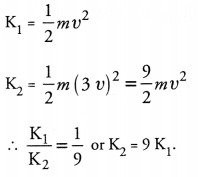Question 11.
Avinash can run with a speed of 8 m s-1 against the frictional force of 10 N, and Kapil can move with a speed of 3 m s-1 against the frictional force of 25 N. Who is more powerful and why ?
P = Fu
Power of Avinash = 10 x 8 = 80 W
Power of Kapil = 25 x 3 = 75 W
So, Avinash is more powerful.

Question 12.
A boy is moving on a straight road against a frictional force of 5 N. After travelling a distance of 1.5 km he forgot the correct path at a round about (Fig. 1) of radius 100 m.However, he moves on the circular path for one and half cycle and then he moves forward upto 2.0 km. Calculate the work done by him.
Here, F = 5N, Distance travelled S = 1500 + 3 π r + 2000 = 4442.86 m
W = F x S = 5 x 4442.86 = 22214.3 J.

Question 13.
Can any object have mechanical energy even if its momentum is zero ? Explain.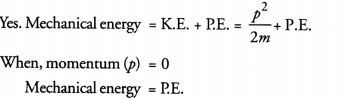Question 14.
Can any object have momentum even if its mechanical energy is zero ? Explain.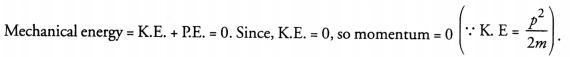Question 15.
The power of a motor pump is 2 kW. How much water per minute the pump can raise to a height of 10 m ? (Given g = 10 m s-2).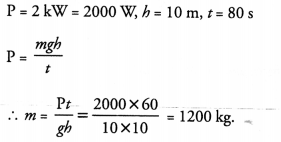Question 16.
The weight of a person on a planet A is about half that on the earth. He can jump upto 0.4 m height on the surface of the earth. How high he can jump on the planet A ?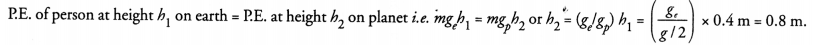Question 17.
The velocity of a body moving in a straight line is increased by applying a constant force F, for some distance in the direction of the motion. Prove that the increase in the kinetic energy of the body is equal to the work done by the force on the body.
Consider a body or an object of mass m moving with velocity u. Let a force F be applied on the body so that the velocity attained by the body after travelling a distance S is v (Figure 5).Work done by the force on the body is given by
W =      FS                                                                               …(i)
Since velocity of the body changes so the body is accelerated. Let a be the acceleration of the body. Therefore, according to Newton’s second law of motion,
F =     ma                                                                             …(ii)
Using eqn. (ii) in eqn. (i), we get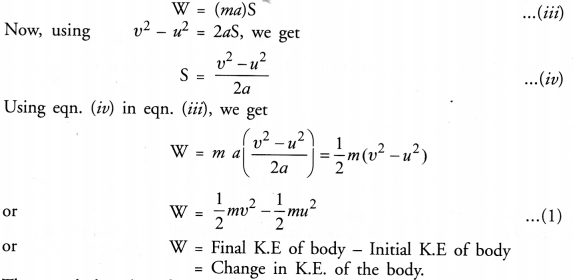Thus, work done by a force on a body is equal to the change in kinetic energy of the body This is known as work-energy theorem.

Question 18.
Is it possible that an object is in the state of accelerated motion due to external force acting on it, but no work is being done by the force. Explain it with an example.
Yes. When body moves in a circular path.

Question 19.
A ball is dropped from a height of 10 m. If the energy of the ball reduces by 40% after striking the ground, how much high can the ball bounce back ? (g = 10 m s-2). (CBSE 2013)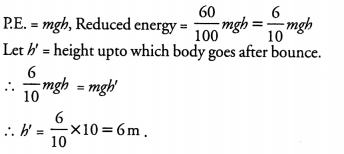Question 20.
If an electric iron of 1200 W is used for 30 minutes everyday, find electric energy consumed in the month of April.
Energy consumed in one day = P x t= 1200 W x ½ h = 600 Wh
Energy consumed in 30 days = 600 Wh x 30 = 1800 Wh =18 kWh.

Question 21.
A light and a heavy object have the same momentum. Find out the ratio of their kinetic energies. Which one has a larger kinetic energy ?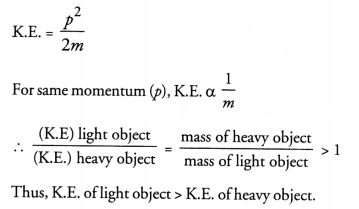Question 22.
An automobile engine propels a 1000 kg car (A) along a levelled road at a speed of 36 km h-1. Find power if the opposing frictional force is 100 N. Now, suppose after travelling a distance of 200 m, this car collides with another stationary car (B) of same mass and comes to rest. Let its engine also stops at the same time. Now car (B) starts moving on the same level road without getting its engine started. Find the speed of car (B) just after the collision.
Force = 100 N, v = 36 km h-1 = 36 x (5/18) = 10 m s-1
Power, P = Force x velocity = 100 x 10 = 1000 W
According to law of conservation of linear momentum
Momentum of car A + Mementum of car B before collision = momentum of car A + momentum of car B after collision
i.e. 1000 x 10 + 1000 x 0 = 1000 x 0 + 1000 x v
v = 10 m s-1
Thus, speed of car B just after collision =10 ms-1

Question 23.
A girl having mass of 35 kg sits on a trolley of mass 5 kg. The trolley is given an initial velocity of 4 ms-1 by applying a force. The trolley comes to rest after travelling a distance of 16m.
(a) How much work is done on the trolley ?
(b) How much work is done by the girl ?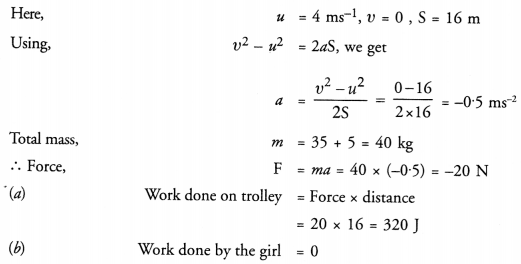Question 24.
Four men lift a 250 kg box to a height of 1 m and hold it without raising or lowering it.
(a) How much work is done by the men in lifting the box ?
(b) How much work do they do in just holding it ?
(c) Why do they get tired while holding it ? (g = 10m s-2)

Question 25.
(a) W = F x S = mgS= 250 x 10 x 1 =25.0 J.
(b) Zero. This is because displacement of box is zero.
(c) They get tired because muscular force applied by them is needed to balance the weight of the box.
The Jog Falls in Karnataka State are nearly 200m high. 2000 tonnes of water falls from it in a minute. Calculate the equivalent power if all this energy can be utilized ? (g = 10 ms-2)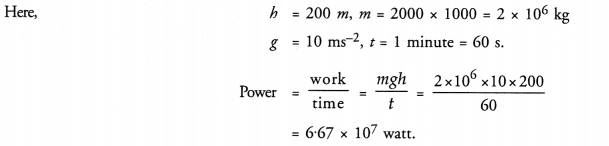Question 26.
How is the power related to the speed at which a body can be lifted ? How many kilograms will a man working at the power of 100 W, be able to lift at constant speed of 1 ms-1 vertically ? (g = 10 ms-2)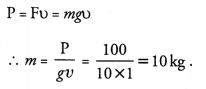Question 27.
Define watt. Express kilowatt in terms of joule per second. A 150 kg car engine develops 500 W for each kg. What force does it exert in moving the car at a speed of 20 m s-1 ?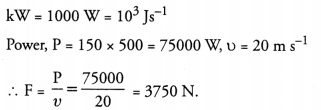Question 28.
Compare the power at which each of the following is moving upwards against the force of gravity ? (given g = 10 ms-2)

1. a butterfly of mass 1.0 g that flies upward at a rate of 0.5 m s-1 .
2. a 250 g squirrel climbing up on a tree at a rate of 0.5 m s-1.